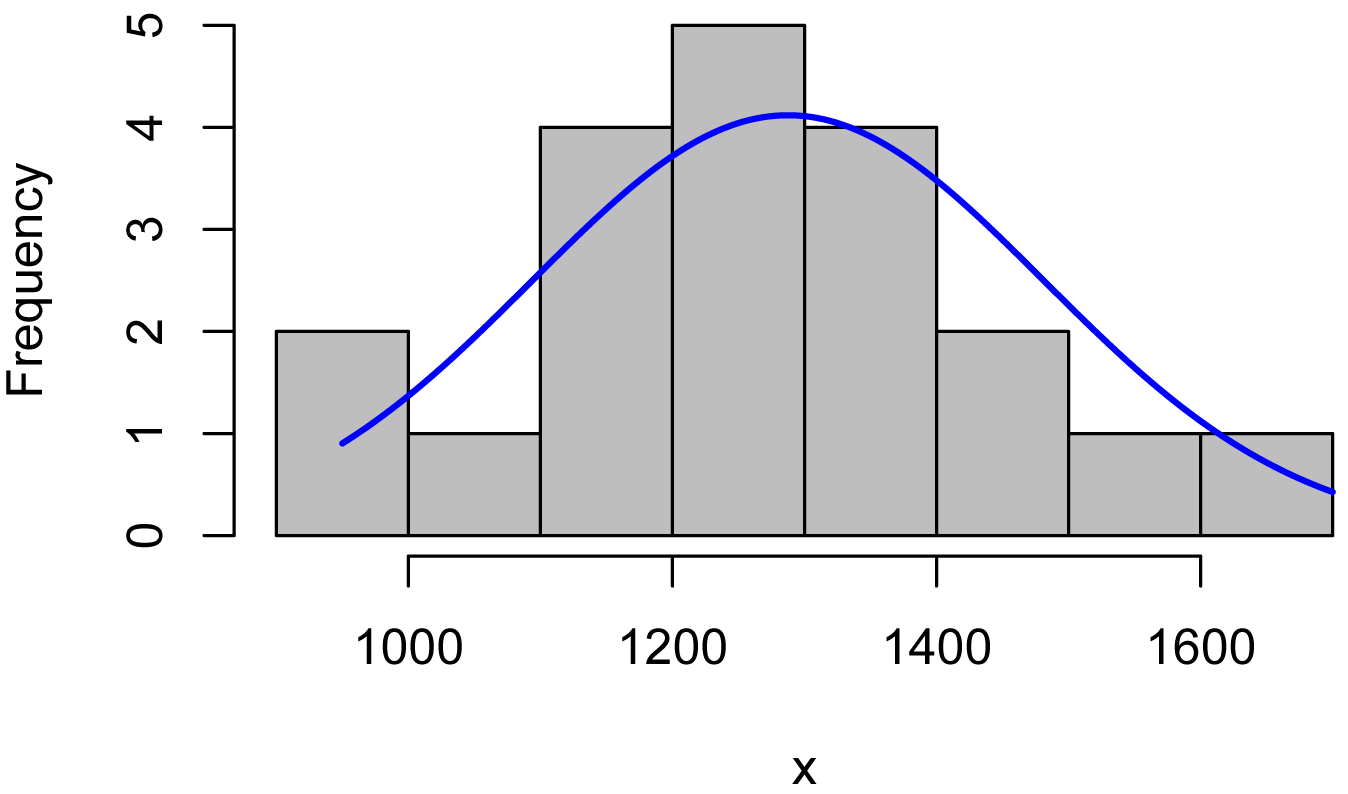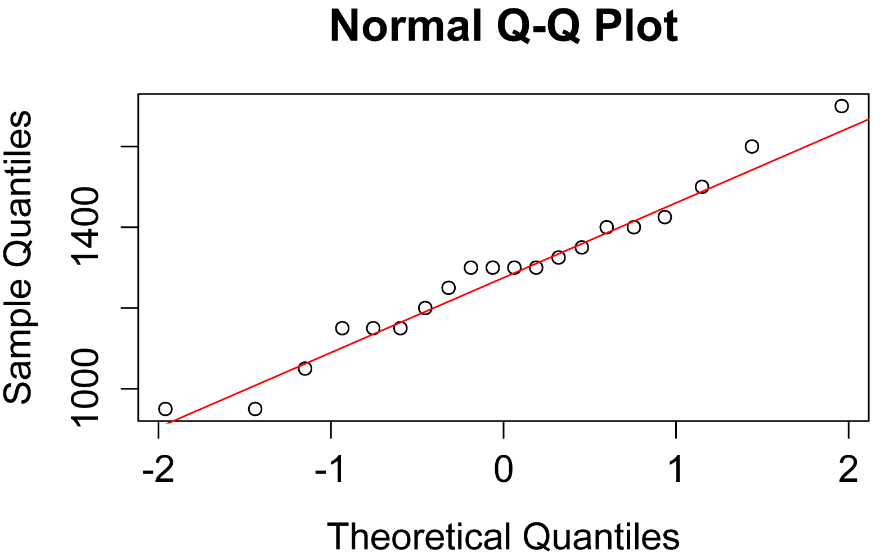## Summary and Analysis of Extension Program Evaluation in R

Salvatore S. Mangiafico

# One-sample t-test

One-sample tests are not used too often, but are useful to compare a set of values to a given default value.  For example, one might ask if a set of student scores are significantly different from a “default” or “neutral” score of 75.

##### Appropriate data

•  One-sample data

•  Data are interval/ratio, and are continuous

•  Data are normally distributed

•  Moderate skewness is permissible if the data distribution is unimodal without outliers

##### Hypotheses

•  Null hypothesis:  The mean of the population from which the data were sampled is equal to the default value.

•  Alternative hypothesis (two-sided): The mean of the population from which the data were sampled is not equal to the default value.

##### Interpretation

Reporting significant results as, e.g., “Mean score for Variable A was significantly different from a default value of 75” is acceptable.

##### Other notes and alternative tests

•  The nonparametric analogue for this test is the one-sample Wilcoxon signed-rank test.

•  Power analysis for the one-sample t-test can be found at Mangiafico (2015) in the References section.

### Packages used in this chapter

The packages used in this chapter include:

•  psych

•  rcompanion

•  lsr

•  ggplot2

The following commands will install these packages if they are not already installed:

if(!require(psych)){install.packages("psych")}
if(!require(rcompanion)){install.packages("rcompanion")}
if(!require(lsr)){install.packages("lsr")}
if(!require(ggplot2)){install.packages("ggplot2")}

### One-sample t-test example

In the following example, Brendon Small has his SNAP-Ed students keep diaries of what they eat for a week, and then calculate the daily sodium intake in milligrams.  As a first step in the analysis, he wants to compare mean sodium intake by his students to the American Heart Association recommendation of 1500 mg.

A one-sample t-test can be conducted with the t.test function in the native stats package.  Conveniently the output includes the mean of the sample, a confidence interval for that mean, and a p-value for the t-test.

We will use a histogram with an imposed normal curve to confirm data are approximately normal.

Input = ("
Instructor       Student  Sodium
'Brendon Small'  a        1200
'Brendon Small'  b        1400
'Brendon Small'  c        1350
'Brendon Small'  d         950
'Brendon Small'  e        1400
'Brendon Small'  f        1150
'Brendon Small'  g        1300
'Brendon Small'  h        1325
'Brendon Small'  i        1425
'Brendon Small'  j        1500
'Brendon Small'  k        1250
'Brendon Small'  l        1150
'Brendon Small'  m         950
'Brendon Small'  n        1150
'Brendon Small'  o        1600
'Brendon Small'  p        1300
'Brendon Small'  q        1050
'Brendon Small'  r        1300
'Brendon Small'  s        1700
'Brendon Small'  t        1300
")

###  Check the data frame

library(psych)

str(Data)

summary(Data)

### Remove unnecessary objects

rm(Input)

#### Histogram of data

A histogram of the data can be examined to determine if the data are sufficiently normal.

x = Data\$Sodium

library(rcompanion)

plotNormalHistogram(x)#### Normal quantile plot of data

x = Data\$Sodium

qqnorm(x)

qqline(x, col="red")#### One-sample t-test

t.test(Data\$Sodium,
mu       = 1500,
conf.int = 0.95)

One Sample t-test

t = -4.9053, df = 19, p-value = 9.825e-05

alternative hypothesis: true mean is not equal to 1500

95 percent confidence interval:
1196.83 1378.17

sample estimates:
mean of x
1287.5

### Effect size

Cohen’s d can be used as an effect size statistic for a one-sample t-test.  It is calculated as the difference between the mean of the data and mu, the default value, all divided by the standard deviation of the data.

It ranges from 0 to infinity, with 0 indicating no effect where the mean equals mu.  In some versions, Cohen’s d can be positive or negative depending on whether the mean is greater than or less than mu.

A Cohen’s d of 0.5 suggests that the mean and mu differ by one-half the standard deviation of the data.  A Cohen’s d of 1.0 suggests that the mean and mu differ by one standard deviation of the data.

#### Interpretation of Cohen’s d

Interpretation of effect sizes necessarily varies by discipline and the expectations of the experiment, but for behavioral studies, the guidelines proposed by Cohen (1988) are sometimes followed.  They should not be considered universal.

 Small Medium Large Cohen’s d 0.2 – < 0.5 0.5 – < 0.8 ≥ 0.8

____________________________

Source: Cohen (1988).

#### Cohen’s d for one-sample t-test

The cohensD function in the lsr package has an option for the one-sample t-test.  The grammar follows that of the t.test function.  Note that this function reports the value as a positive number.

library(lsr)

cohensD(Data\$Sodium,
mu = 1500)

 1.096864

It could also be calculated manually from the mean, mu, and standard deviation.

Mean = mean(Data\$Sodium)

Mean

1287.5

Mu   = 1500

Sd   = sd(Data\$Sodium)

Diff = Mean - Mu

Diff

-212.5

CohenD = (Mean - Mu) / Sd

CohenD

 -1.096864

### Box plot with default value

library(ggplot2)

ggplot(data=Data,
aes(x = Instructor, y = Sodium)) +
geom_boxplot() +
geom_point(aes(x = 1, y = 1500),
colour="blue",
size = 8,
shape = "+") +
theme_bw() +
theme(axis.title = element_text(face = "bold")) +
theme(axis.text = element_text(face = "bold"))Box plot of daily sodium intake in milligrams recorded in student diaries.  The blue cross indicates the American Heart Association recommendation of 1500 mg.

### References

Cohen, J. 1988. Statistical Power Analysis for the Behavioral Sciences, 2nd Edition. Routledge.

“Student’s t–test for One Sample” in Mangiafico, S.S. 2015. An R Companion for the Handbook of Biological Statistics, version 1.09. rcompanion.org/rcompanion/d_01.html.

### Exercises O

1. Considering Brendon Small’s data,

a.  What was the mean sodium intake?

b.  Is the data distribution reasonably normal?

c.  Was the mean sodium intake significantly different from the American Heart Association recommendation or 1500 mg per day?

d.  What do you conclude practically? Include a description of the difference between the mean of the data and the American Heart Association recommendation. If they’re different, which is higher? Include effect size, any other relevant summary statistics, and your practical conclusions.

2.  As part of a professional skills program, a 4-H club tests its members for typing proficiency.  Dr. Katz wants to test his students’ mean typing speed against a nominal speed of 40 words per minute.

Instructor                             Student  Words.per.minute
'Dr. Katz Professional Therapist'      a        35
'Dr. Katz Professional Therapist'      b        50
'Dr. Katz Professional Therapist'      c        55
'Dr. Katz Professional Therapist'      d        60
'Dr. Katz Professional Therapist'      e        65
'Dr. Katz Professional Therapist'      f        60
'Dr. Katz Professional Therapist'      g        70
'Dr. Katz Professional Therapist'      h        55
'Dr. Katz Professional Therapist'      i        45
'Dr. Katz Professional Therapist'      j        55
'Dr. Katz Professional Therapist'      k        60
'Dr. Katz Professional Therapist'      l        45
'Dr. Katz Professional Therapist'      m        65
'Dr. Katz Professional Therapist'      n        55
'Dr. Katz Professional Therapist'      o        50
'Dr. Katz Professional Therapist'      p        60

For each of the following, answer the question, and show the output from the analyses you used to answer the question.

a.  What was the mean typing speed?

b.  Is the data distribution reasonably normal?

c.  Was the mean typing speed significantly different from the nominal rate of 40 words per minute?

d.  What do you conclude practically? Include a description of the difference between the mean of the data and the 40 words per minute standard. If they’re different, which is higher? Include effect size, any other relevant summary statistics, and your practical conclusions.